Test: Arithmetic Reasoning- 1

# Test: Arithmetic Reasoning- 1

Test Description

## 25 Questions MCQ Test Logical Reasoning (LR) and Data Interpretation (DI) | Test: Arithmetic Reasoning- 1

Test: Arithmetic Reasoning- 1 for SSC CGL 2022 is part of Logical Reasoning (LR) and Data Interpretation (DI) preparation. The Test: Arithmetic Reasoning- 1 questions and answers have been prepared according to the SSC CGL exam syllabus.The Test: Arithmetic Reasoning- 1 MCQs are made for SSC CGL 2022 Exam. Find important definitions, questions, notes, meanings, examples, exercises, MCQs and online tests for Test: Arithmetic Reasoning- 1 below.
Solutions of Test: Arithmetic Reasoning- 1 questions in English are available as part of our Logical Reasoning (LR) and Data Interpretation (DI) for SSC CGL & Test: Arithmetic Reasoning- 1 solutions in Hindi for Logical Reasoning (LR) and Data Interpretation (DI) course. Download more important topics, notes, lectures and mock test series for SSC CGL Exam by signing up for free. Attempt Test: Arithmetic Reasoning- 1 | 25 questions in 25 minutes | Mock test for SSC CGL preparation | Free important questions MCQ to study Logical Reasoning (LR) and Data Interpretation (DI) for SSC CGL Exam | Download free PDF with solutions
 1 Crore+ students have signed up on EduRev. Have you?
Test: Arithmetic Reasoning- 1 - Question 1

### The total of the ages of Amar, Akbar and Anthony is 80 years. What was the total of their ages three years ago ?

Detailed Solution for Test: Arithmetic Reasoning- 1 - Question 1

Required sum = (80 - 3 x 3) years = (80 - 9) years = 71 years.

Test: Arithmetic Reasoning- 1 - Question 2

### Two bus tickets from city A to B and three tickets from city A to C cost Rs. 77 but three tickets from city A to B and two tickets from city A to C cost Rs. 73. What are the fares for cities B and C from A ?

Detailed Solution for Test: Arithmetic Reasoning- 1 - Question 2

Let Rs. x be the fare of city B from city A and Rs. y be the fare of city C from city A.

Then, 2x + 3y = 77 ...(i) and

3x + 2y = 73 ...(ii)

Multiplying (i) by 3 and (ii) by 2 and subtracting, we get: 5y = 85 or y = 17.

Putting y = 17 in (i), we get: x = 13.

Test: Arithmetic Reasoning- 1 - Question 3

### A number of friends decided to go on a picnic and planned to spend Rs. 96 on eatables. Four of them, however, did not turn up. As a consequence, the remaining ones had to contribute Rs. 4 each extra. The number of those who attended the picnic was

Detailed Solution for Test: Arithmetic Reasoning- 1 - Question 3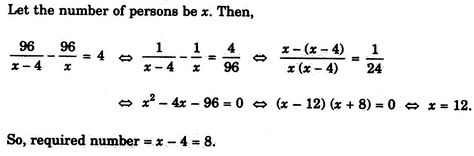Test: Arithmetic Reasoning- 1 - Question 4

An institute organised a fete and 1/5 of the girls and 1/8 of the boys participated in the same. What fraction of the total number of students took part in the fete ?

Detailed Solution for Test: Arithmetic Reasoning- 1 - Question 4

Ans is "Data inadequate".

Suppose ther are 18 students (10 girls, 8 boys).
1/5 of 10=2 (girls).
1/8 of 8=1 (boy).
Total participation=2+1=3.
Total students=10+8=18.
Answer = 3/18 = 1/6.
Answer cannot be determined until we have the "Ratio of girls to boys"

Test: Arithmetic Reasoning- 1 - Question 5

A, B, C, D and E play a game of cards. A says to B, "If you give me three cards, you will have as many as E has and if I give you three cards, you will have as many as D has." A and B together have 10 cards more than what D and E together have. If B has two cards more than what C has and the total number of cards be 133, how many cards does B have ?

Detailed Solution for Test: Arithmetic Reasoning- 1 - Question 5

Clearly, we have :

B-3 = E ...(i)

B + 3 = D ...(ii)

A+B = D + E+10 ...(iii)

B = C + 2 ...(iv)

A+B + C + D + E= 133 ...(v)

From (i) and (ii), we have : 2 B = D + E ...(vi)

From (iii) and (vi), we have : A = B + 10 ...(vii)

Using (iv), (vi) and (vii) in (v), we get:

(B + 10) + B + (B - 2) + 2B = 133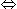5B = 125B = 25.

Test: Arithmetic Reasoning- 1 - Question 6

A pineapple costs Rs. 7 each. A watermelon costs Rs. 5 each. X spends Rs. 38 on these fruits. The number of pineapples purchased is

Detailed Solution for Test: Arithmetic Reasoning- 1 - Question 6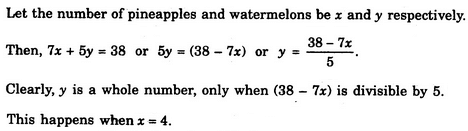Test: Arithmetic Reasoning- 1 - Question 7

A woman says, "If you reverse my own age, the figures represent my husband's age. He is, of course, senior to me and the difference between our ages is one-eleventh of their sum." The woman's age is

Detailed Solution for Test: Arithmetic Reasoning- 1 - Question 7

Let x and y be the ten's and unit's digits respectively of the numeral denoting the woman's age.
Then, woman's age = (10x + y) years; husband's age = (10y + x) years.

Therefore (10y + x)- (10X + y) = (1/11) (10y + x + 10x + y)
⇒ (9y-9x) = (1/11)(11y + 11x) = (x + y)
⇒ 10x = 8yx = (4/5)y

Clearly, y should be a single-digit multiple of 5, which is 5.
So, x = 4, y = 5.
Hence, woman's age = 10x + y = 45 years.

Test: Arithmetic Reasoning- 1 - Question 8

A girl counted in the following way on the fingers of her left hand : She started by calling the thumb 1, the index finger 2, middle finger 3, ring finger 4, little finger 5 and then reversed direction calling the ring finger 6, middle finger 7 and so on. She counted upto 1994. She ended counting on which finger ?

Detailed Solution for Test: Arithmetic Reasoning- 1 - Question 8

Clearly, while counting, the numbers associated to the thumb will be : 1, 9,17, 25,.....

i.e. numbers of the form (8n + 1).

Since 1994 = 249 x 8 + 2, so 1993 shall correspond to the thumb and 1994 to the index finger.

Test: Arithmetic Reasoning- 1 - Question 9

A man has Rs. 480 in the denominations of one-rupee notes, five-rupee notes and ten-rupee notes. The number of notes of each denomination is equal. What is the total number of notes that he has ?

Detailed Solution for Test: Arithmetic Reasoning- 1 - Question 9

Let number of notes of each denomination be x.

Then x + 5x + 10x = 480

⇒ 16x = 480

∴ x = 30.

Hence, total number of notes = 3x = 90.

Test: Arithmetic Reasoning- 1 - Question 10

What is the product of all the numbers in the dial of a telephone ?

Detailed Solution for Test: Arithmetic Reasoning- 1 - Question 10

Since one of the numbers on the dial of a telephone is zero, so the product of all the numbers on it is 0.

Test: Arithmetic Reasoning- 1 - Question 11

A is 3 years older to B and 3 years younger to C, while B and D are twins. How many years older is C to D?

Detailed Solution for Test: Arithmetic Reasoning- 1 - Question 11

Since B and D are twins, so B = D.
Now, A = B + 3 and A = C - 3.
Thus, B + 3 = C - 3
⇒ D + 3 = C-3
⇒ C - D = 6

Test: Arithmetic Reasoning- 1 - Question 12

The 30 members of a club decided to play a badminton singles tournament. Every time a member loses a game he is out of the tournament. There are no ties. What is the minimum number of matches that must be played to determine the winner ?

Detailed Solution for Test: Arithmetic Reasoning- 1 - Question 12

Clearly, every member except one (i.e. the winner) must lose one game to decide the winner. Thus, minimum number of matches to be played = 30 - 1 = 29.

Test: Arithmetic Reasoning- 1 - Question 13

In a garden, there are 10 rows and 12 columns of mango trees. The distance between the two trees is 2 metres and a distance of one metre is left from all sides of the boundary of the garden. The length of the garden is

Detailed Solution for Test: Arithmetic Reasoning- 1 - Question 13

Each row contains 12 plants.

There are 11 gapes between the two corner trees (11 x 2) metres and 1 metre on each side is left.

Therefore Length = (22 + 2) m = 24 m.

Test: Arithmetic Reasoning- 1 - Question 14

12 year old Manick is three times as old as his brother Rahul. How old will Manick be when he is twice as old as Rahul ?

Detailed Solution for Test: Arithmetic Reasoning- 1 - Question 14

Manick's present age = 12 years, Rahul's present age = 4 years.

Let Manick be twice as old as Rahul after x years from now.
Then, 12 + x = 2 (4 + x)
⇒ 12 + x = 8 + 2x
⇒ x = 4.

Hence, Manick's required age = 12 + x = 16 years.

Test: Arithmetic Reasoning- 1 - Question 15

A tailor had a number of shirt pieces to cut from a roll of fabric. He cut each roll of equal length into 10 pieces. He cut at the rate of 45 cuts a minute. How many rolls would be cut in 24 minutes ?

Detailed Solution for Test: Arithmetic Reasoning- 1 - Question 15

Number of cuts made to cut a roll into 10 pieces = 9.

Therefore Required number of rolls = (45 x 24)/9 = 120.

Test: Arithmetic Reasoning- 1 - Question 16

In a class of 60 students, the number of boys and girls participating in the annual sports is in the ratio 3 : 2 respectively. The number of girls not participating in the sports is 5 more than the number of boys not participating in the sports. If the number of boys participating in the sports is 15, then how many girls are there in the class ?

Detailed Solution for Test: Arithmetic Reasoning- 1 - Question 16

Let the number of boys and girls participating in sports be 3x and 2x respectively.
Then, 3x = 15 or x = 5
⇒ Number of girls participating in sports = 2x = 10
⇒  Number of students not participating in sports = 60 - (15 + 10) = 35

Let number of boys not participating in sports be y.
Then, number of girls not participating in sports = (35 -y)

Therefore (35 - y) = y + 5
⇒  2y = 30
⇒  y = 15

So, number of girls not participating in sports = (35 - 15) = 20

Hence, total number of girls in the class = (10 + 20) = 30

Test: Arithmetic Reasoning- 1 - Question 17

There are deer and peacocks in a zoo. By counting heads they are 80. The number of their legs is 200. How many peacocks are there ?

Detailed Solution for Test: Arithmetic Reasoning- 1 - Question 17

Let x and y be the number of deer and peacocks in the zoo respectively. Then,

x + y = 80 ...(i) and

4x + 2y = 200 or 2x + y = 100 ...(ii)

Solving (i) and (ii), we get) x = 20, y = 60.

Test: Arithmetic Reasoning- 1 - Question 18

A man wears socks of two colours - Black and brown. He has altogether 20 black socks and 20 brown socks in a drawer. Supposing he has to take out the socks in the dark, how many must he take out to be sure that he has a matching pair ?

Detailed Solution for Test: Arithmetic Reasoning- 1 - Question 18

Since there are socks of only two colours, so two out of any three socks must always be of the same colour.

Test: Arithmetic Reasoning- 1 - Question 19

A motorist knows four different routes from Bristol to Birmingham. From Birmingham to Sheffield he knows three different routes and from Sheffield to Carlisle he knows two different routes. How many routes does he know from Bristol to Carlisle ?

Detailed Solution for Test: Arithmetic Reasoning- 1 - Question 19

Total number of routes from Bristol to Carlisle = (4 x 3 x 2) = 24.

Test: Arithmetic Reasoning- 1 - Question 20

Mac has £ 3 more than Ken, but then Ken wins on the horses and trebles his money so that he now has £ 2 more than the original amount of money that the two boys had between them. How much money did Mac and Ken have between them before Ken's win?

Detailed Solution for Test: Arithmetic Reasoning- 1 - Question 20

Let money with Ken = x. Then, money with Mac = x + £ 3.

Now, 3x = (x + x + £ 3) + £ 2 ⇒ x = £ 5.

Therefore Total money with Mac and Ken = 2x + £ 3 = £ 13.

Test: Arithmetic Reasoning- 1 - Question 21

In a class, there are 18 boys who are over 160 cm tall. If these constitute three-fourths of the boys and the total number of boys is two-thirds of the total number of students in the class, what is the number of girls in the class ?

Detailed Solution for Test: Arithmetic Reasoning- 1 - Question 21

Let the number of boys be x. Then, (3/4)x = 18 or x = 18 x(4/3) = 24.

If total number of students is y, then (2/3) y = 24 or y = 24 x (3/2) = 36.

Therefore Number of girls in the class = (36 - 24) = 12.

Test: Arithmetic Reasoning- 1 - Question 22

A father is now three times as old as his son. Five years back, he was four times as old as his son. The age of the son (in years) is

Detailed Solution for Test: Arithmetic Reasoning- 1 - Question 22

Let son's age be x years. Then, father's age = (3x) years.
Five years ago, father's age = (3x - 5) years and son's age = (x - 5) years.

So, 3x - 5 = 4 (x - 5)
⇒ 3x - 5 = 4x - 20
⇒  x = 15

Test: Arithmetic Reasoning- 1 - Question 23

A waiter's salary consists of his salary and tips. During one week his tips were 5/4 of his salary. What fraction of his income came from tips ?

Detailed Solution for Test: Arithmetic Reasoning- 1 - Question 23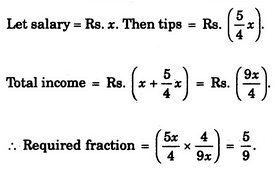Test: Arithmetic Reasoning- 1 - Question 24

If you write down all the numbers from 1 to 100, then how many times do you write 3 ?

Detailed Solution for Test: Arithmetic Reasoning- 1 - Question 24

Clearly, from 1 to 100, there are ten numbers with 3 as the unit's digit- 3, 13, 23, 33, 43, 53, 63, 73, 83, 93; and ten numbers with 3 as the ten's digit - 30, 31, 32, 33, 34, 35, 36, 37, 38, 39.

So, required number = 10 + 10 = 20.

Test: Arithmetic Reasoning- 1 - Question 25

If 100 cats kill 100 mice in 100 days, then 4 cats would kill 4 mice in how many days ?

Detailed Solution for Test: Arithmetic Reasoning- 1 - Question 25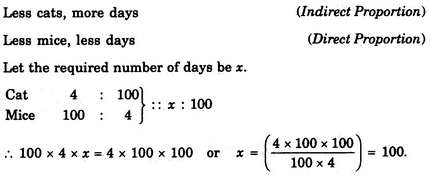## Logical Reasoning (LR) and Data Interpretation (DI)

96 videos|83 docs|119 tests
 Use Code STAYHOME200 and get INR 200 additional OFF Use Coupon Code
Information about Test: Arithmetic Reasoning- 1 Page
In this test you can find the Exam questions for Test: Arithmetic Reasoning- 1 solved & explained in the simplest way possible. Besides giving Questions and answers for Test: Arithmetic Reasoning- 1, EduRev gives you an ample number of Online tests for practice

## Logical Reasoning (LR) and Data Interpretation (DI)

96 videos|83 docs|119 tests